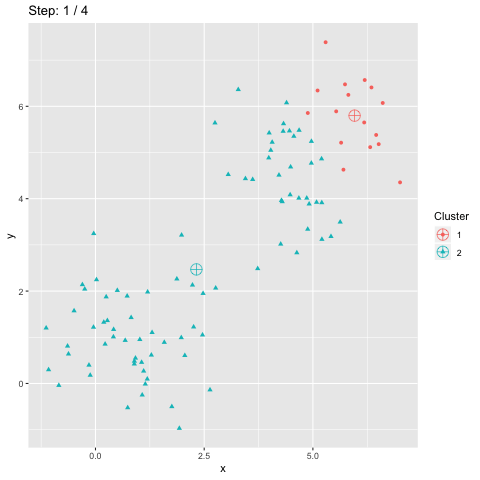# Blog Archives

## Animate intermediate results of your algorithm

February 19, 2019
ByThe R package gganimate enables to animate plots. It is particularly interesting to visualize the intermediate results of an algorithm, to see how it converges towards the final results. The following illustrates this with K-means clustering. The outline of this post is as follows: We will first generate some artificial data to work with. This allows to visualize the behavior of the algorithm....

## Animate intermediate results of your algorithm

February 19, 2019
ByThe R package gganimate enables to animate plots. It is particularly interesting to visualize the intermediate results of an algorithm, to see how it converges towards the final results. The following illustrates this with K-means clustering. The outline of this post is as follows: We will first generate some artificial data to work with. This allows to visualize the behavior of the algorithm....

## Chaining effect in clustering

January 21, 2019
ByIn a previous blog post, I explained how we can leverage the k-means clustering algorithm to count the number of red baubles on a Christmas tree. This method fails however if we put Christmas tinsels on it. Let’s find a solution for this more difficult case. Filter red points Let's first proceed as we did for Christmas baubles by filtering the...

## How many red Christmas baubles on the tree?

January 5, 2019
ByChristmas time is over. It is time to remove the Cristmas tree. But just before removing it, one can ask: How many red Christmas baubles are on the tree? In order to answer this question, we will proceed with the following steps: Transform the picture into a dataframe, which is more convenient to handle. Filter the red points from the others. Group the red points...

## Gaussian mixture models: k-means on steroids

December 22, 2018
ByThe k-means algorithm assumes the data is generated by a mixture of Gaussians, each having the same proportion and variance, and no covariance. These assumptions can be alleviated with a more generic algorithm: the CEM algorithm applied on a mixture of Gaussians. To illustrate this, we will first apply a more generic clustering algorithm than k-means on nine synthetic datasets previously...

## K-means is not all about sunshines and rainbows

December 9, 2018
ByK-means is the most known and used clustering algorithm. It has however several drawbacks and does not behave nicely on some datasets. In fact, every clustering algorithm has its own strenghts and drawbacks. Each relies on some assumptions on the dataset and leverages these properties to cluster the data into groups. The No Free Lunch Theorem states that "any two algorithms are equivalent...

## Generate datasets to understand some clustering algorithms behavior

November 11, 2018
ByIn order to understand how a clustering algorithm works, good sample datasets are useful to highlight its behavior under certain circumstances. This post shows how to generate 9 datasets: a mixture of two Gaussians with same size, variance and no covariance, Gaussians which differ only from their means and sizes, Gaussians which differ only from their means and variances, Gaussians with a different...